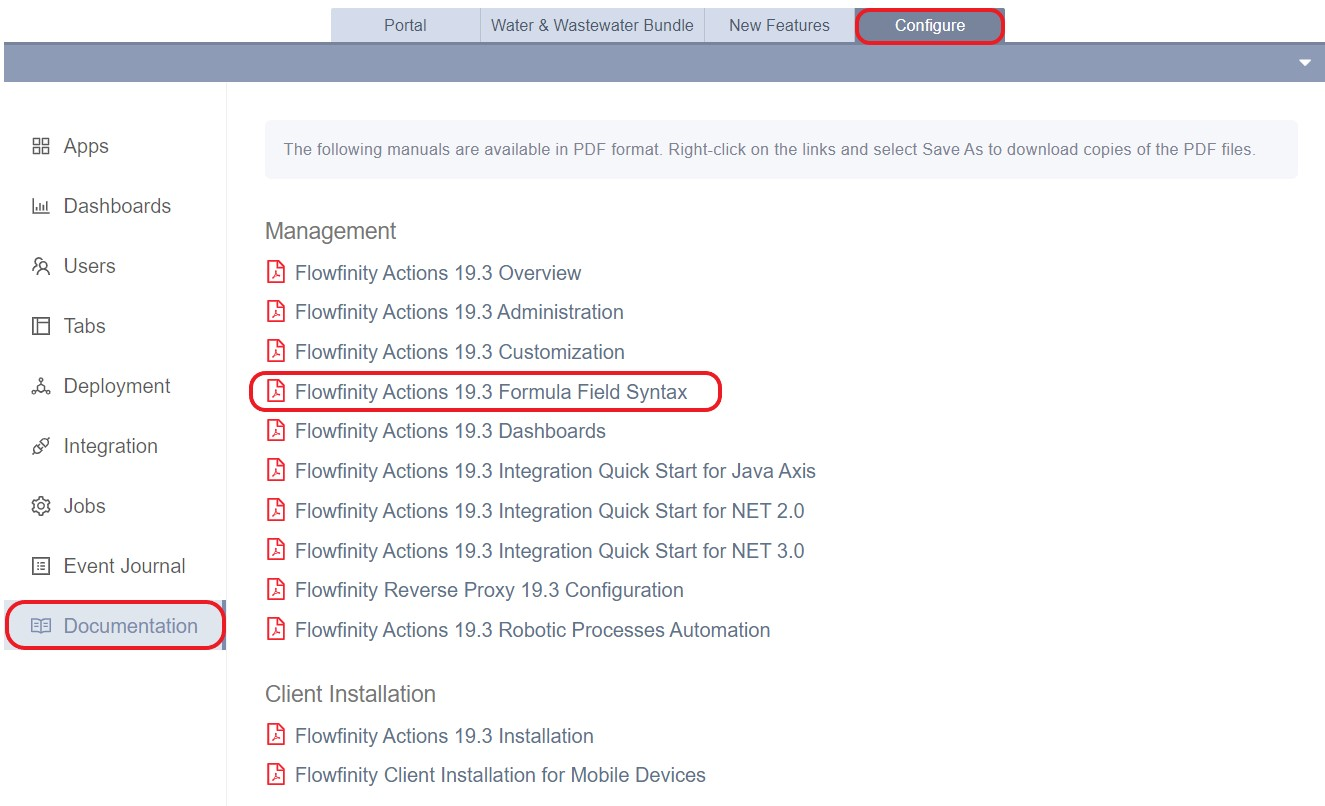﻿ Introduction to calculations and variable text | Flowfinity Knowledge Base

# Calculations and variable text introduction

In Flowfinity, you can use formulas to carry out simple or complex calculations and logic. Some examples include adding up labor and parts costs in a work order, calculating a total score for an inspection or audit, or combining several text fields into a single message.

Formulas are written declaratively in the application editor, and can either be calculated all the time, or once as a default value for a field. Most field types can use formulas, and there are functions available to work with and convert different types of field values: date, numeric, and text.

Formulas can be calculated while the user is online or offline without a network connection.​

Here are some key things to know:

• You can use your data fields in a formula by typing Control + Spacebar and selecting them from a list, or typing in the "Name" of the field.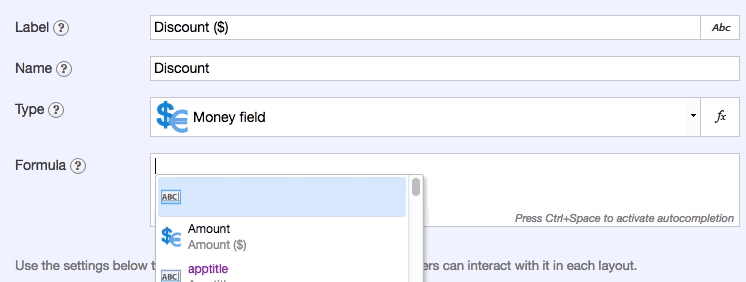Example: Multiply product amount and percent discount to get the discount in dollars
• Logic and conditions can be used in formula calculations.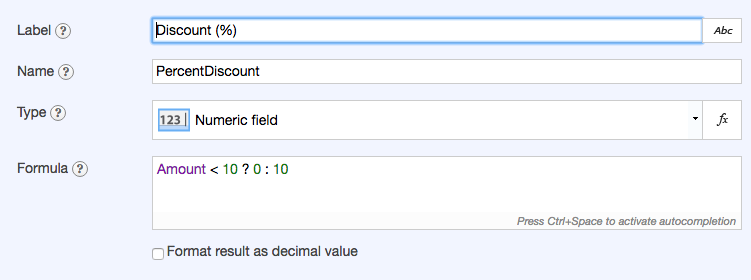Example: If the product amount is less than \$10, the discount is zero, otherwise it is 10%
• More than 100 pre-defined functions are available to use in formulas, such as sum, max or min, date/time operations, locations, and more.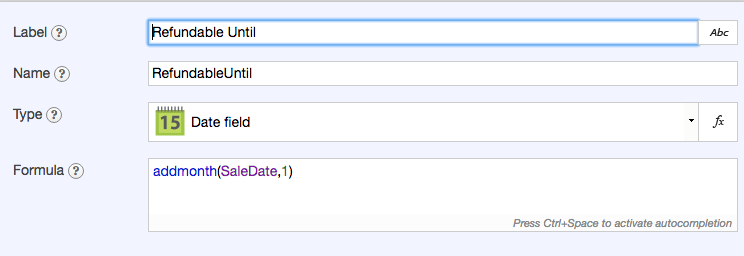Example: Calculate one month after the sales date, as the last date that the item can be refunded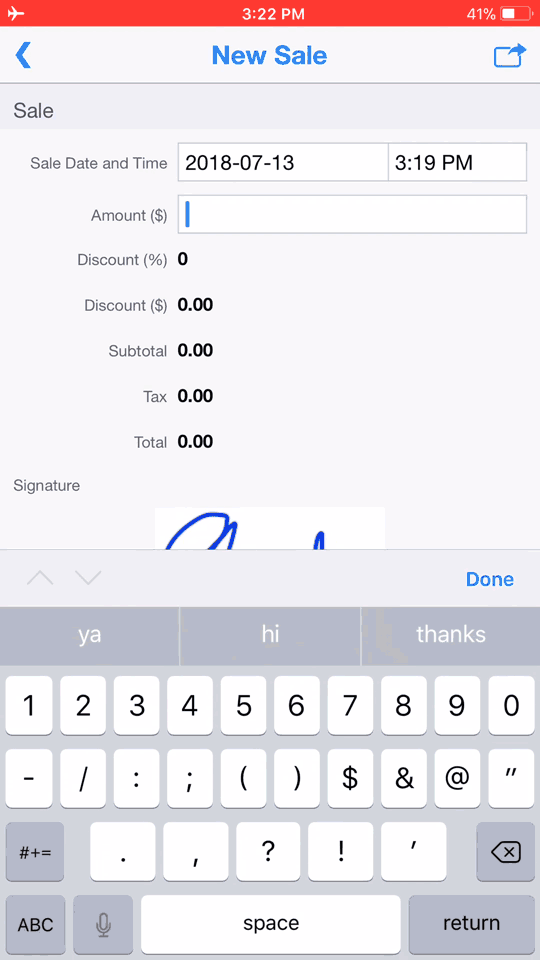Example: If the sale amount is less than \$10.00, no discount is applied. If it's \$10.00 or greater, a 10% discount is applied. The last date for a refund is calculated as a month later than the sale date and time.

These are some simple examples of how you can use Flowfinity formulas. For a complete guide, please refer to the "Formula Field Syntax" documentation located in the Configure tab, Documentation section.